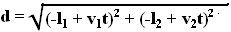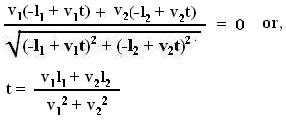## Sunday, July 15, 2007

### Irodov Problem 1.16The position of the particle moving along the horizontal line in the Figure is given by -l1 + v1t and that of the particle moving in the vertical direction is given by -l2 + v2t. As shown in the figure the distance between the particle is given by,The minimum can be obtained by differentiating d with respect to t and equating it to 0. Thus,The distance for nearest approach can be obtained by substituting the value of t in the expression for d and obtained as,